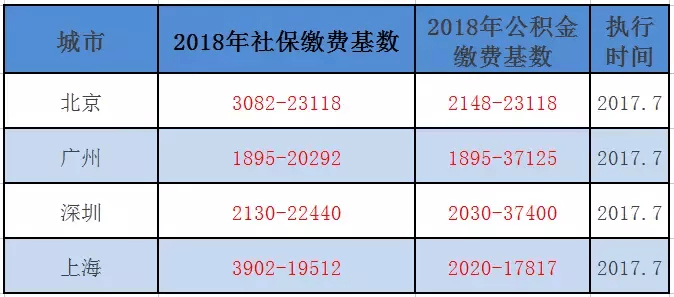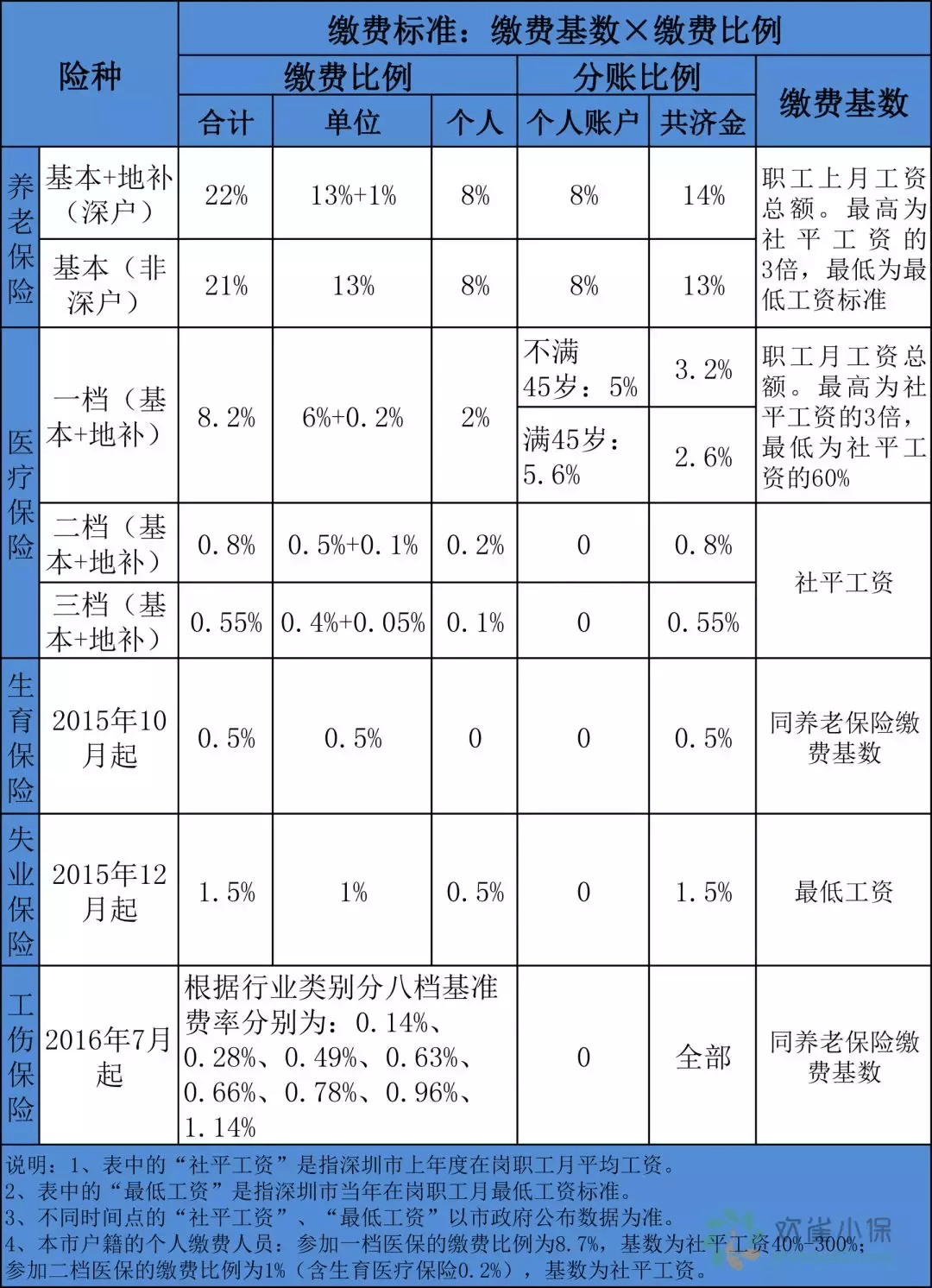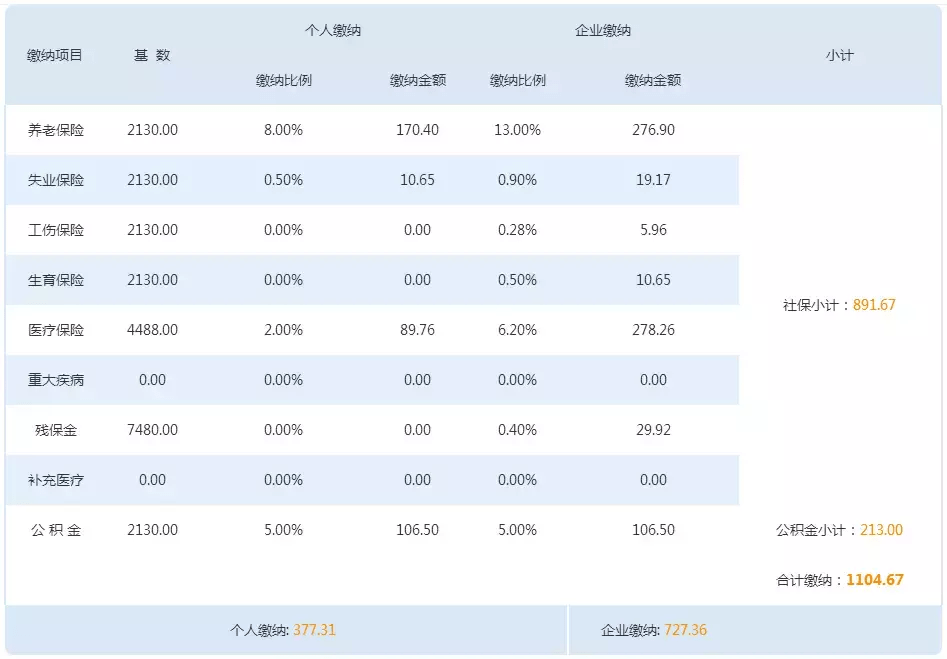# 五险一金扣多少（五险一金怎么扣费呢）

“为什么一年下来

“五险一金到底是怎么扣费的呢？”

“每个月扣多少钱？”

「每月你的五险一金都是怎么扣费的」」

2018年各地社保基数表（注：以上仅选取部分城市作示例）【个人月缴纳养老保险】=2130*8.00%=170.40（元）

【个人月缴纳失业保险】=2130*0.50%=10.65（元）

【个人月缴纳工伤保险】=2130*0.00%=0（元）

【个人月缴纳生育保险】=2130*0.00%=0（元）

【个人月缴纳医疗保险】=4488*2.00%=89.76（元）

【个人月缴纳住房公积金】=2130*5.00%=106.50（元）

【个人月缴纳五险一金】=170.40+10.65+89.76+106.50=377.31（元）• QQ咨询
• 回顶